Many GRE math problems involve 2D shapes, which include triangles, squares, and in general, the kind of shapes you might remember seeing in first grade. It turns out these shapes actually have a number of interesting properties, knowledge of which matters for doing well on the GRE. These shapes are often defined by the number of sides they have. Now, there is no 2D shape that is made of just 1 line:And similarly, there is no 2D shape with just 2 lines: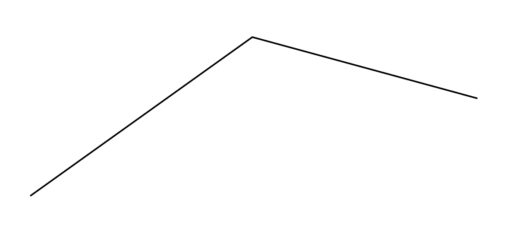But starting with three lines, we get: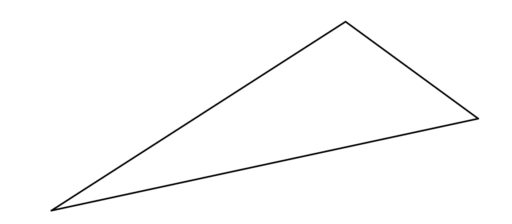No doubt you know that this is called a triangle. With four lines we get the general category of quadrilaterals (or four-sided shapes):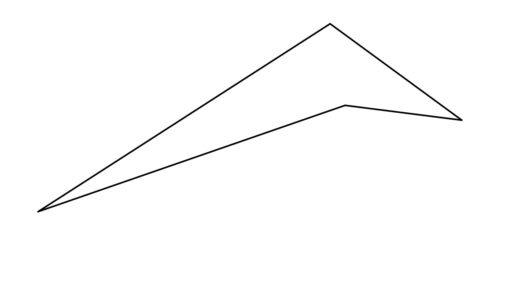This category includes trapezoids, squares, rectangles, parallelograms, and rhombuses: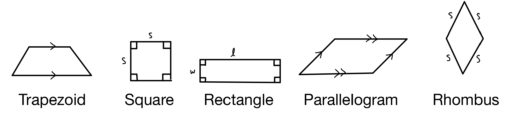(The arrows above indicate that those two lines are parallel to one another).

And we can also have a 2D shape defined by a constant distance from a central point: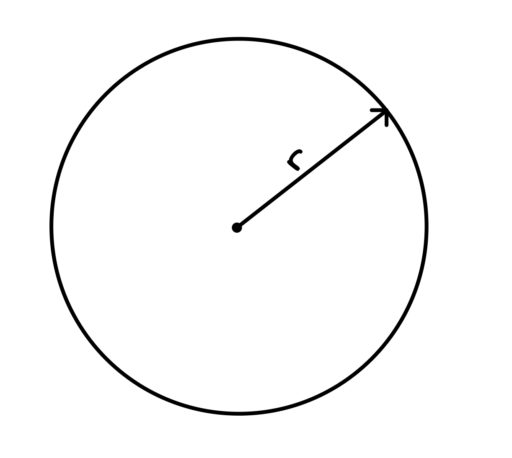and we call this a circle.

Now, of courses there are other 2D shapes; we can have pentagons (5 sides), hexagons (6 sides), and more. (A chiliagon has 1000 sides). But for the GRE, we will generally need just the above.

Finally, we also need the idea of a regular polygon.

Regular Polygon: Regular polygons have sides of equal length and angles of equal degree.

So, for example, the regular 3-sided polygon is the equilateral triangle: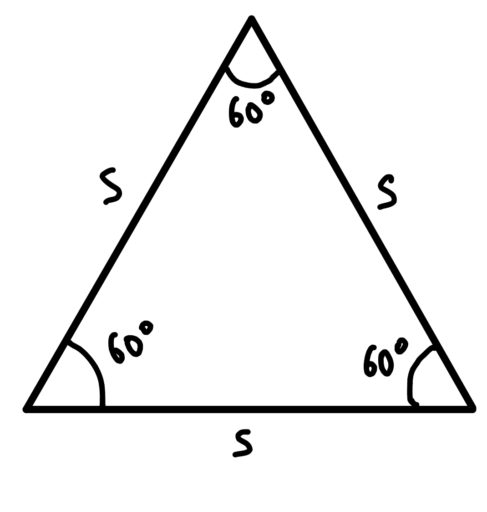which, as the name equi-lateral suggests, has three sides of equal length. And its angles are all 60 degrees.

The regular polygon for four sides is the square: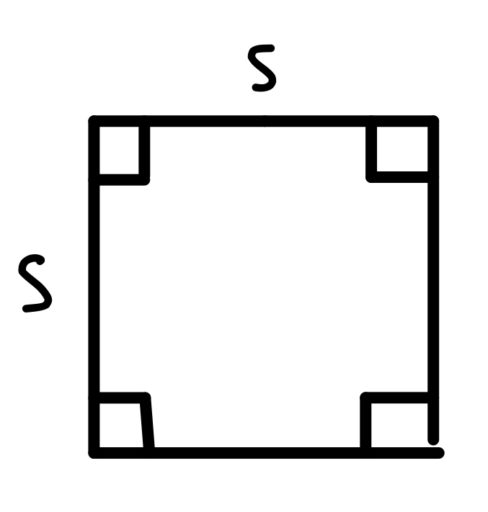where each angle is 90 degrees.

You may have noticed a pattern here: 60 degrees for 3 sides; 90 degrees for 4 sides. And this pattern holds for any number of sides:

Angles of a Regular Polygon
If we have a regular polygon ofsides, each angle isdegrees.

This formula can be important when trying to find certain angle values; for example:

Example 1
You have a seven-sided regular polygon. Give the degree of an angle of the polygon.

Example 2
You have a regular octagon. What is the sum of all of the angles of the octagon?

And the fact that regular polygons have sides of equal length can help in problems involving the perimeter of a regular polygon:

Example 3
A regular 29-sided polygon has a perimeter of 116. What is the length of its sides?